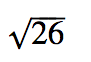Rational Numbers

My Definition

Key Characteristics

The following are true of rational numbers:

• Rational numbers can be expressed as the ratio of two integers
p q
where q ≠ 0

• Terminating decimals are rational numbers.

• Repeating decimals are rational numbers.

Example

Use the link below to further study examples of rational numbers.

Rational Numbers Activity

Non-exampleThis is not a rational number. It cannot be written as the ratio of two integers, where the denominator is not equal to zero.

The decimal representation is neither a terminating decimal nor a repeating decimal.

TEKS: 6(2)(A), 8(2)(A)